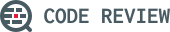## Don't touch my junk.

0

(click on this box to dismiss)Q&A for peer programmer code reviews

``````CREATE TABLE #Numbers
(
Number DECIMAL(10,4),
Variance DECIMAL(10,4)
);
GO
INSERT INTO #Numbers (Number) VALUES
(23),
(37),
(45),
(49),
(56),
(63),
(63),
(70),
(72),
(82);
GO

DECLARE @Mean DECIMAL(10,4);
SET @Mean = (SELECT SUM (Number) / COUNT(Number) FROM #Numbers);
-- @Mean is 100
UPDATE #Numbers
SET Variance = POWER( (@Mean - Number), 2);
DECLARE @StdVariance DECIMAL(10,4);
SET @StdVariance = (SELECT SQRT( SUM((1.0 * Number - @Mean) * (1.0 * Number - @Mean)) / COUNT(Number) ) FROM #Numbers);
SELECT
@Mean AS [Mean],
@StdVariance AS [Standard Variance],
Avg(Number) as [Avg],
StDev(Number) as [StdDev]
from #Numbers``````

### Enter Parameters

Options:
-Hold tight while we fetch your results
:records returned in :time ms:cached